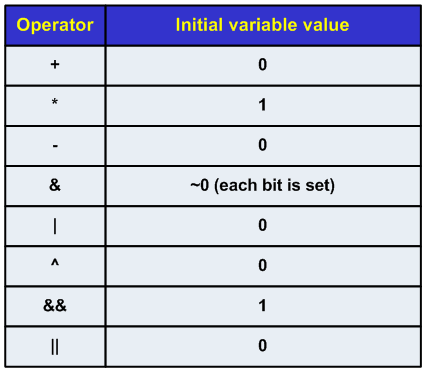﻿ Parallel notes N5 - continuing to study OpenMP constructs# Parallel notes N5 - continuing to study OpenMP constructs

Here is the next post devoted to studying the parallel programming technology OpenMP. Here we will consider two directives: atomic, reduction.

## atomic directive

Consider this code that sums up array items:

``````intptr_t A, sum = 0;
for (intptr_t i = 0; i < 1000; i++)
A[i] = i;
for (intptr_t i = 0; i < 1000; i++)
sum += A[i];
printf("Sum=%Ii\n", sum);``````

The result is:

``````Sum=499500
Press any key to continue . . .``````

Let us try to parallelize this code using the directives "omp" and "parallel":

``````#pragma omp parallel for
for (intptr_t i = 0; i < 1000; i++)
sum += A[i];``````

Unfortunately, this way of parallelization is incorrect because a race condition occurs in this code. Several threads try to access the variable "sum" for reading and writing simultaneously. The access order might be the following:

``````Value of "sum" variable = 500;
Value of "i" in the first thread = 1;
Value of "i" in the second thread = 501;
Thread 1: processor register = sum
Thread 2: processor register = sum
Thread 1: processor register += i
Thread 2: processor register += i
Thread 2: sum = processor register
Thread 1: sum = processor register
The value of "sum" variable is 501, not 1002.``````

You may also make sure that this kind of parallelization is incorrect by launching the demo code. What I got was, in particular, this:

``````Sum=486904
Press any key to continue . . .``````

To avoid the errors of refreshing shared variables, you may use critical sections. However, if the variable "sum" is shared while the operator has the form sum=sum+expr, it is more convenient to use "atomic" directive. "atomic" directive is faster than critical sections because some atomic operations can be directly replaced by processor commands.

This directive refers to the assignment operator that follows it straight and guarantees correct processing of the shared variable standing on its left side. While this operator is being executed, accesses from all the threads being launched at the moment are locked except for the thread that executes the operation.

The directive atomic applies only to operations of this type:

• X BINOP= EXPR
• X++
• ++X
• X−−
• −−X

Here X is scalar variable, EXPR is an expression with scalar types that lacks the variable X, BINOP is a non-overloaded operator +, *, -, /, &, ^, |, <<, >>. You must not use the directive "atomic" in any other case.

The corrected version of the code looks as follows:

``````#pragma omp parallel for
for (intptr_t i = 0; i < 1000; i++)
{
#pragma omp atomic
sum += A[i];
}``````

This solution gives a correct result but is quite ineffective. The speed of this code is lower than that of the serial version. There will constantly appear locks when the algorithm is running, and as a result, the work of the cores will be nothing but mere waiting. "atomic" directive is used in this sample only to demonstrate how it works. In practice, it is reasonable to use this directive when accesses to shared variables are rare. For example:

``````unsigned count = 0;
#pragma omp parallel for
for (intptr_t i = 0; i < N; i++)
{
if (SlowFunction())
{
#pragma omp atomic
count++;
}
}``````

Remember that in an expression the directive "atomic" is applied to, only processing of the variable on the left side of the assignment operator is atomic while the calculations on its right side are not necessarily such. Let us study this by an example where the directive "atomic" in no way influences the call of the functions participating in the expression:

``````class Example
{
public:
unsigned m_value;
Example() : m_value(0) {}
unsigned GetValue()
{
return ++m_value;
}
unsigned GetSum()
{
unsigned sum = 0;
#pragma omp parallel for
for (ptrdiff_t i = 0; i < 100; i++)
{
#pragma omp atomic
sum += GetValue();
}
return sum;
}
};``````

This sample contains a race condition and the result it returns may vary from run to run. The directive "atomic" in this code protects increment of the variable "sum". But "atomic" directive does not influence the call of the function GetValue(). The calls are performed in concurrent threads and it leads to errors when trying to perform the operation "++m_value" inside the function GetValue.

## reduction directive

It would be logical to ask how one can quickly sum up the array items. The answer is - with the help of reduction directive.

The directive's format: reduction(operator:list)

Possible operators - "+", "*", "-", "&", "|", "^", "&&", "||".

List serves to list the names of the shared variables. These must have a scalar type (for example, float, int or long, but not std::vector, int [], etc.).

The working principle:

• Local copies are created in each thread for each variable.
• The local copies are initialized according to the operator's type. For additive operations it is 0 or its analogs, for multiplicative ones it is 1 or its analogs. See also Table N1.
• When all the parallel region's operators are executed, the defined operator is executed to process the local copies of the variables. The order of operator execution is not defined.Now the effective code that uses reduction looks as follows:

``````#pragma omp parallel for reduction(+:sum)
for (intptr_t i = 0; i < 1000; i++)
sum += A[i];``````

To be continued in the next issue of "Parallel Notes"...##### Use PVS-Studio to search for bugs in C, C++, C# and Java

We offer you to check your project code with PVS-Studio. Just one bug found in the project will show you the benefits of the static code analysis methodology better than a dozen of the articles.

411
14 123

### Do you make errors in the code?